## SUMPRODUCT

The SUMPRODUCT function returns the sum of the products of corresponding numbers in one or more arrays.

SUMPRODUCT (array 1, array 2, …array 30)

array1: A array  of cells. array 1  is a reference to a single range of cells containing values of any type. If string or Boolean values
array 2,……. array 30: Optionally include one or more additional array ( ranges) of cells. The ranges must all have the same dimensions.

Usage Notes
The SUMPRODUCT function multiplies the corresponding numbers in each range and then sums each of the products. If only one range is specified, SUMPRODUCT returns the sum of the range.

In it’s traditional form or classic form, SUMPRODUCT multiplies each value in one array by the corresponding value in another array, and returns the summed result. As an example, if cells A1:A3 contain the values 1,2,3 and B1:B3 contain 10,20,30, then

=SUMPRODUCT(A1:A3,B1:B3)

returns 140, or (1*10)+(2*20)+(3*30)=10+40+90=140.

This is a useful function, but nothing more than that. A further, more ‘creative’ use of SUMPRODUCT has evolved, and is still evolving. This has been a creative and productive process that has significantly increased the useability of SUMPRODUCT,

There are two very useful functions that support conditional counting and summing, namely COUNTIF and SUMIF. Very useful functions, but limitation in that they can only evaluate a single test range.

To know more about the countif function have look at the following link
https://anandexcels.wordpress.com/2012/01/28/countif/

To know more about the Sumif have look at the following link
https://anandexcels.wordpress.com/2012/01/28/sumif-function/

The only constrain in these function is it will evaluate a single criteria, all thou we can force them to do multiple criteria using IF function

We will look into more rational way of forcing he sumproduct function to do more using a array formula. if you observe in the functions list it is under the array formula’s group. lets try to understand the functionality using an example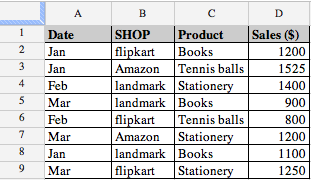We can easily count the number of Amazon with
=countif(B2:B9,”Amazon”)
which returns 2.
Similalrly, it is straight-forward to get the value of products sold through “Amazon”, using
=SUMIF(B2:B9,”Amazon”,D2:D9)
which gives 2725.
But supposing that we want a count of how many Amazon  sold in Jan, or the value of them? The number can be calculated with
=ArrayFormula(SUM(IF(B2:B9=”Amazon”,IF(A2:A9=”Jan”,1,0),0)))
which is an array formula. Similarly, the value is obtained with
=ArrayFormula(SUM(IF(B2:B9=”Amazon”,IF(A2:A9=”Jan”,D2:D9,0),0)))
which is also an array formula.

But as this page is about SUMPRODUCT, you would expect that we could use that function in this case, and we can. The solution for the number of Amazon sold in Jan using this function is
=ArrayFormula(SUMPRODUCT((B2:B9=”Amazon”)*(A2:A9=”Jan”)))
The value is obtained with
=ArrayFormula(SUMPRODUCT((B2:B9=”Amazon”)*(A2:A9=”Jan”)*(D2:D9)))
The * is being used as the AND operator, the formula is saying, where A2:A9 = Amazon AND B2:B9 = Jan, and where A2:A9 = Amazon AND B2:B9= Jan, multiplied by D2:D9.

This is relatively easy to use when there are multiple criteria.

We can see that the * is equivalent to AND in the formula, how this works is explained later, but supposing we want an OR condition. As a further extension of its use, we use the ‘+’ (plus) operator to count OR conditions, such as how many books sold were either
Flipkart or Landmark. The formula for this is
=ArrayFormula(SUMPRODUCT((B2:B9=”Flipkart”)+(B2:B9=”landmark”)))
which returns the result 6 as expected
So far, so good, in that we have a versatile function that can do any number of conditional tests, and has an inbuilt flexibility that provides extensibility.

## SUMPRODUCT Explained

Understanding how SUMPRODUCT works helps to determine where to use it, how to can construct thus formula, and thus how it can be extended. below shows an example data set that we will use.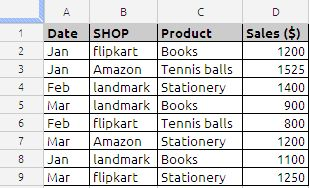In this example, the problem is to find how many Amazon with a date in Jan were sold. A2:A9 holds the Date, B2:B9 has the Shop, and D2:D9 has the number sold. The formula to get this result is
=ArrayFormula(SUMPRODUCT((B2:B9=”Amazon”)*(A2:A9=”Jan”)*(D2:D9)))
The first part of the formula (B2:B9=”Amazon”) checks the array of makes for a value of Amazon. This returns an array of TRUE/FALSE, in this case it is
{FALSE,TRUE,FALSE,FALSE,FALSE,TRUE,FALSE,FALSE}
Similarly, the categories are checked for the vale Date with (A2:A9=”Jan”). Again, this returns an array of TRUE/FALSE, or
{TRUE,TRUE,FALSE,FALSE,FALSE,FALSE,TRUE,FALSE}
And finally, the numbers are not checked but taken as is, that is (D2:D9), which returns an array of numbers
{1200,1525,1400,900,800,1200,1100,1250}
So now we have three arrays, two of TRUE/FALSE values, one of numbers. This is showm in below.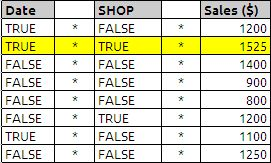And this is where it gets interesting.

SUMPRODUCT usually works on arrays of numbers, but we have arrays of TRUE/FALSE values as well as an array of numbers. By using the ‘*’ (multiply) operator, we can get numeric values that can be summed. ‘*’ has the effect of coercing these two arrays into a single array of 1/0 values. Multiplying TRUE by TRUE returns 1 (try it, enter =TRUE()*TRUE() in a cell and see the result), any other combination returns 0. Therefore, when both conditions are satisfied, we get a 1, whereas if any or both conditions are not satisfied, we get a 0. Multiplying the first array of TRUE/FALSE values by the second array of TRUE/FALSE values returns a composite array of 1/0 values, or

{0,1,0,0,0,0,0,0}*{1200,1525,1400,900,800,1200,1100,1250}
which will result in 1525

Similarly we can count the same
=ArrayFormula(SUMPRODUCT((B2:B9=”Amazon”)*(A2:A9=”Jan”)))
the arrays will be as show in the below example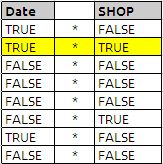and after executing the above formulas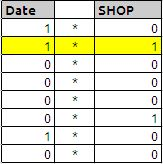and answer is highlighted in yellow colour which will result in value 1

If you have been able to follow this explanation all of the way through, it may have occurred to you that although we are using the SUMPRODUCT function, the ‘*’ operators have resolved the multiple arrays into a single composite array, leaving SUMPRODUCT to simply sum the members of that composite array, that is, there is no product. This is perfectly correct, and perfectly valid,SUMPRODUCT can work on a single array (put 1,2,3 in cells A1,A2,A3, and insert =SUMPRODUCT(A1:A3) in a cell, it returns 6 correctly). In reality, we only need the ‘*’ to coerce the arrays that are being tested for a particular condition, we do not need it for the array that is not subject to a conditional test. So we could also use

=ArrayFormula(SUMPRODUCT((B2:B9=”Amazon”)*(A2:A9=”Jan”)*(D2:D9)))

which does use the product aspect .